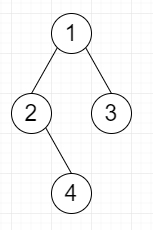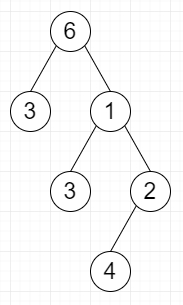# Inverted Subtree in C++

Suppose we have two binary trees called source and target; we have to check whether there is some inversion T of source such that it is a subtree of the target. So, it means there is a node in target that is identically same in values and structure as T including all of its descendants.

As we know a tree is said to be an inversion of another tree if either −

• Both trees are empty

• Its left and right children are optionally swapped and its left and right subtrees are inversions.

So, if the input is like sourceTargetthen the output will be True

To solve this, we will follow these steps −

• Define a function check(), this will take node1, node2,

• if node1 and node2 both are null, then −

• return true

• if node1 or node2 any one of them is null, then −

• return false

• if val of node1 is not equal to val of node2, then −

• return false

• op1 := check(left of node1, left of node2) and check(right of node1, right of node2)

• op2 := check(right of node1, left of node2) and check(left of node1, right of node2)

• return true when at least one of op1 and op2 is true

• Define a function solve(), this will take source, target,

• if source and target are empty, then −

• return true

• if source or target any one of them is null, then −

• return false

• op1 := check(target, source)

• if op1 is true, then −

• return true

• return true when at least one of the solve(source, left of target) or solve(source, right of target) is true

Let us see the following implementation to get better understanding −

## Example

Live Demo

#include <bits/stdc++.h>
using namespace std;
class TreeNode {
public:
int val;
TreeNode *left, *right;
TreeNode(int data) {
val = data;
left = NULL;
right = NULL;
}
};
class Solution {
public:
bool check(TreeNode* node1, TreeNode* node2){
if(!node1 && !node2)
return true;
if(!node1 || !node2)
return false;
if(node1->val != node2->val) {
return false;
}
bool op1 = check(node1->left, node2->left) && check(node1->right, node2->right);
bool op2 = check(node1->right, node2->left) && check(node1->left, node2->right);
return op1 || op2;
}
bool solve(TreeNode* source, TreeNode* target) {
if(!target && !source)
return true;
if(!target || !source)
return false;
bool op1 = check(target, source);
if(op1)
return true;
return solve(source, target->left) || solve(source, target->right);
}
};
main(){
Solution ob;
TreeNode *target = new TreeNode(6);
target->left = new TreeNode(3);
target->right = new TreeNode(1);
target->right->left = new TreeNode(3);
target->right->right = new TreeNode(2);
target->right->right->left = new TreeNode(4);
TreeNode *source = new TreeNode(1);
source->left = new TreeNode(2);
source->right = new TreeNode(3);
source->left->right = new TreeNode(4);
cout << (ob.solve(source, target));
}

## Input

TreeNode *target = new TreeNode(6);
target->left = new TreeNode(3);
target->right = new TreeNode(1);
target->right->left = new TreeNode(3);
target->right->right = new TreeNode(2);
target->right->right->left = new TreeNode(4);
TreeNode *source = new TreeNode(1);
source->left = new TreeNode(2);
source->right = new TreeNode(3);
source->left->right = new TreeNode(4);

## Output

1